Checkout JEE MAINS 2022 Question Paper Analysis : Checkout JEE MAINS 2022 Question Paper Analysis :

# Oblique Sketch

The oblique sketching is a pictorial representation of an object, in which the diagram is intended to depict the perspective of objects in three dimensions. This type of drawing can be done either in a normal, cabinet or cavalier style. This kind of sketching is generally used by the marketing departments in their business process to illustrate the product and its features. In this article, let us discuss what is an oblique sketch, the difference between the oblique and the isometric sketch, and the procedure to perform the oblique sketching with examples.

## What is Oblique Sketch?

In our day to day life, we come across multiple three-dimensional objects such as prisms, basketball, football etc. A three-dimensional object can be easily represented on a two dimensional plane with the help of an oblique sketch.

Look at the image of the cube given below: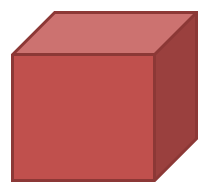We know a cube is a three-dimensional object but the image of cube drawn above on the paper gives us a clear picture as of how a cube looks like. The plane of paper is two dimensional but a three dimensional solid such as the cube can be represented on it. The important point to note here is that not all the faces of the cube are visible to us but we can still figure out the image as a cube. Also, if measured the lengths of all sides drawn in the above image are varying but still the image depicts a cube. Such a representation is known as an oblique sketch.

In the oblique sketching method, the circular lines which are parallel to the front plane of the projection are drawn true shape and size. So, in this case, the circular features appear as a circle, not as an ellipse. This is the main characteristic feature of the oblique sketch. Here, the three axes of the oblique sketches are horizontal, vertical, and at a receding angle that can vary between 30 degrees and 60 degrees.

## Difference Between the Oblique Sketch and Isometric Sketch

The major difference between the isometric and the oblique sketching/drawing are given here.

 Difference Between Isometric and Oblique Sketch Oblique Sketch Isometric Sketch An oblique sketch has a more focus on the front side of an object or the face. Isometric Sketch focuses on the edge of an object. It is drawn usually using the 45-degrees angle to render the third dimensions. It is usually drawn using 30 degrees angles.

### Procedure for Oblique Sketching – Example

An oblique sketch can be defined as a diagram intended to portray the perspective of an object in three dimensions.

Let us try to draw an oblique sketch of a 4 × 4 × 4 cube on a paper.

1. For sketching a 4 × 4 × 4 cube take a squared paper as shown below: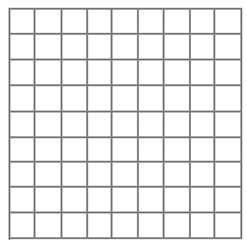2.  Sketch the front face of a cube measuring 4 × 4 as shown below: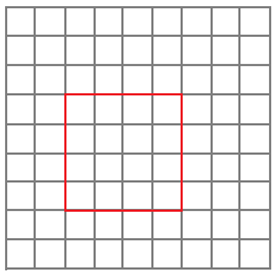3. Sketch the back face which is offset with respect to the front face.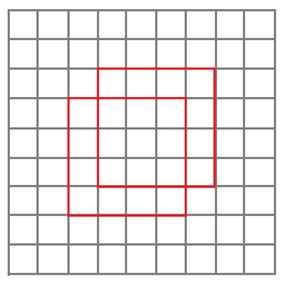4. Join the corresponding corners as shown: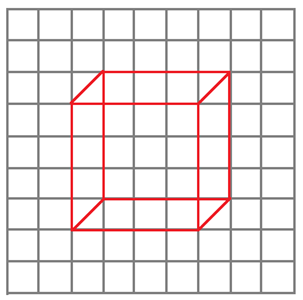5. Join the corresponding corners as shown:6. According to the convention redraw the hidden edges as dotted lines as shown: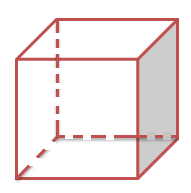Our oblique sketch representing a cube of dimension 4 × 4 × 4 is ready. The following points must be kept in mind while drawing an oblique sketch:

1. Size of the front and back end of the oblique sketch are same
2. All the edges in the cube are equal which also appear in the image but its measurement is different.